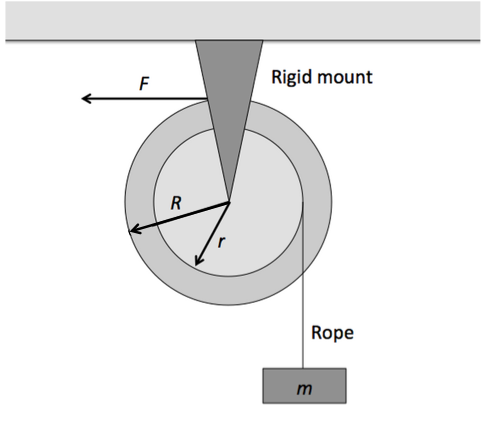Classical Mechanics

# Torque - Dynamical Behavior

The position of a $3 \text{ kg}$ particle moving on the $xy$-plane at time $t$ (in seconds) is given by the equation $\vec{r} = \left( 2 t^2 \hat{i} - (4 t + 4 t^2) \hat{j} \right) \text{ m} .$ Considering the origin $O$ as the pivot, what is the magnitude of the torque on the particle at $t= 6 \text{ s}?$A fixed pulley-like device is used to lift a box of mass $m=13\text{ kg},$ as shown in figure above. The outer radius of the device is $R=7 \text{ m},$ and the radius of the hub is $r= 2 \text{ m}.$ When a constant horizontal force $F = 130 \text{ N}$ is applied to a rope wrapped around the outer rim of the device, the box hanging from a rope wrapped around the hub acquires an upward acceleration of magnitude $2 \text{ m/s}^2.$ Then what is the rotational inertia of the device about its axis of rotation?

The gravitational acceleration is $g= 10 \text{ m/s}^2.$

A particle moves clockwise in a circle around the origin on the $xy$-plane. If its angular momentum as a function of time $t$ (in seconds) is $5 t^2 \text{ kg} \cdot \text{m}^2\text{/s},$ what is the magnitude of the torque on the particle at $t = 4 \text{ s}?$

A $6 \text{ kg}$ particle that moves on the $xy$-plane with velocity $\vec{v} = \left( 6 \hat{i} + 2 \hat{j} \right) \text{ m/s}$ is at $x= 9 \text{ m}$ and $y= 12 \text{ m}.$ It is being pulled by a $6 \text{ N}$ force in the $-x$ direction. Considering the origin as the pivot, what is the torque of the force?

A cubic dice is sliding on a frictionless table. The dice is a cube with edges of length 1 cm and a mass of 30 g. A kid reaches down and gives a horizontal flick to the dice, causing it to change which face is up. What is the minimum impulse in g~cm/s the kid must give to the dice in order to change the face?

Details and assumptions

• The acceleration of gravity is $-9.8~m/s^2$.
• You can model the dice as a perfect cube of uniform density.
• The dice never leave the table
×